## 程序员最近都爱上了这个网站  程序员们快来瞅瞅吧！  it98k网:it98k.com

出租广告位,需要合作请联系站长

+关注

## 前提准备

mysql的安装这里就不再赘述了，大家可以参考其他的模块进行安装

``````pip install pymysql
``````

## 连接数据库

``````
import pymysql
# 连接数据库
# 创建数据库的游标
cursor = db.cursor()
#execute()方法并执行 SQL 语句
cursor.execute("select version()")
# 读取第一条数据
data = cursor.fetchone()
print(data)
# 关闭连接
db.close()

# 输出:
# ('8.0.24',)
``````

• host 数据库ip地址，如果是本地可以用localhost或127.0.0.1 如果是远程就需要指定正确的ip地址
• user 用户名
• port 端口号 如果不指定就默认是3306

cursor()：获取数据库的操作游标
execute() 执行SQL语句，把要进操作的内容写成SQL语句，
fetchone() 读取一条数据
close() 断开连接，释放资源
“select version()” sql语句的执行结果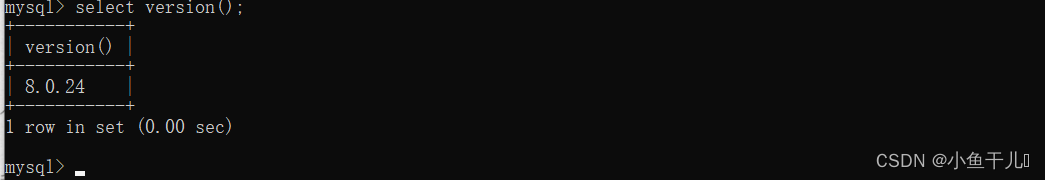## 创建数据库

``````import pymysql
# 连接数据库
# 创建数据库的游标
cursor = db.cursor()
# 创建数据库spiders
cursor.execute("create database spiders")
# 关闭连接
db.close()
``````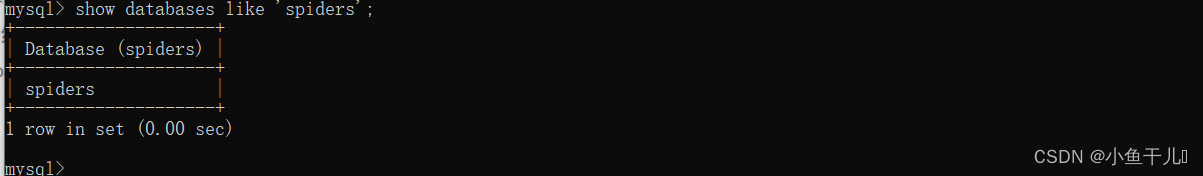## 创建数据表、

``````import pymysql
# 连接数据库
# 创建数据库的游标
cursor = db.cursor()
sql = "create table if not exists students(id varchar(255) not null,name varchar(255) not null,age int not null,primary key (id))"
cursor.execute(sql)

db.close()
``````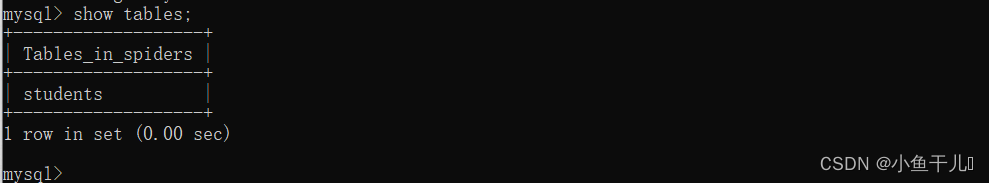## 插入数据

``````import pymysql

cursor = db.cursor()
id = '10005'
name = 'zhangsan'
age = '20'
#方式1
# sql = "insert into students(id,name,age) values('"+id+"','"+name+"','"+age+"')"
# cursor.execute(sql)
# 方式2
# sql = "insert into students(id,name,age) values('{}','{}','{}')".format(id,name,age)
# cursor.execute(sql)
# 方式3（推荐）
sql = "insert into students(id,name,age) values(%s,%s,%s)"
cursor.execute(sql,(id,name,age))
db.commit()
db.close()
``````

## 查询数据

``````import pymysql

try:
sql = 'select * from students'
cursor = db.cursor()
cursor.execute(sql)
d1 = cursor.fetchone()
print("获取一条数据",d1)
all_d = cursor.fetchall()
print("获取所有数据",all_d)
# sql = "insert into students(id,name,age) values('"+id+"','"+name+"','"+age+"')"
except:
print("Error")
db.close()
``````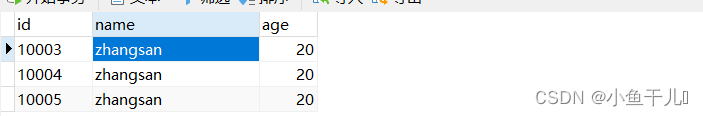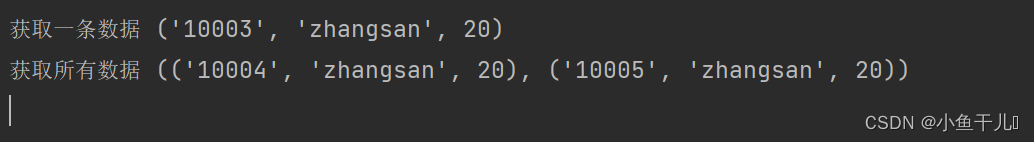``````import pymysql

try:
sql = 'select * from students where id>10003'
cursor = db.cursor()
cursor.execute(sql)
all_d = cursor.fetchall()
print("获取所有数据",all_d)
# sql = "insert into students(id,name,age) values('"+id+"','"+name+"','"+age+"')"
except:
print("Error")
db.close()
``````## 更新数据

``````import pymysql

try:
sql = 'update students set name=%s where id=%s'
cursor = db.cursor()
cursor.execute(sql,('李四','10004'))
db.commit()
except:
db.rollback()
db.close()
``````

## 删除数据

``````import pymysql

try:
sql = 'delete from students where id<%s'
cursor = db.cursor()
cursor.execute(sql,('10004'))
db.commit()
except:
db.rollback()
db.close()

``````

## 实战应用

``````import pymysql

data = {
'id':'10006',
'name':'王五',
'age':45
}
# 连接数据库
table = 'students' # 表名
keys = ','.join(data.keys()) # 拼接插入的字段
values = ','.join(['%s']*len(data)) # 添加占位符
# 使用with关键字，Python 解释器会自动释放资源。 它还提供错误处理
with db:
cursor = db.cursor()
sql = 'insert into {}({}) values({}) on duplicate key update'.format(table, keys, values)
update = ','.join([" {key}=%s".format(key=key) for key in data])
sql += update
try:
cursor.execute(sql, tuple(data.values()) * 2)
db.commit()
except:
db.rollback()

``````

• 0 主键存在，且数据一样没有更新
• 1 主键不存在，插入数据
• 2 主键存在，更新操作

## 总结17 0

pdf(new) 更多>